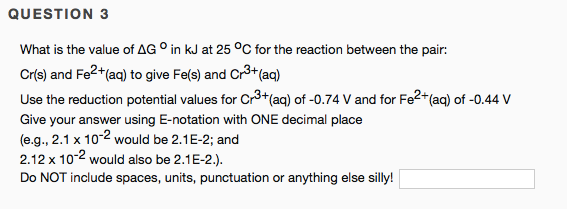# What is the value of ΔG° in kJ at 25 degrees C for the reaction between the pair: Cr(s) and Fe^2+(aq) to give Fe(s) and Cr^3+(aq) Use the reduction potential values for Cr^3+(aq) of -0.74 V and for Fe^2+(aq) of -0.44 V Give your answer using E-notation with ONE decimal place (e.g., 2.1 x 10^-2 would be 2.1 E-2; and 2.12 x 10^-2 would also be 2.1 E-2). Do NOT include spaces, units, punctuation or anything else silly!